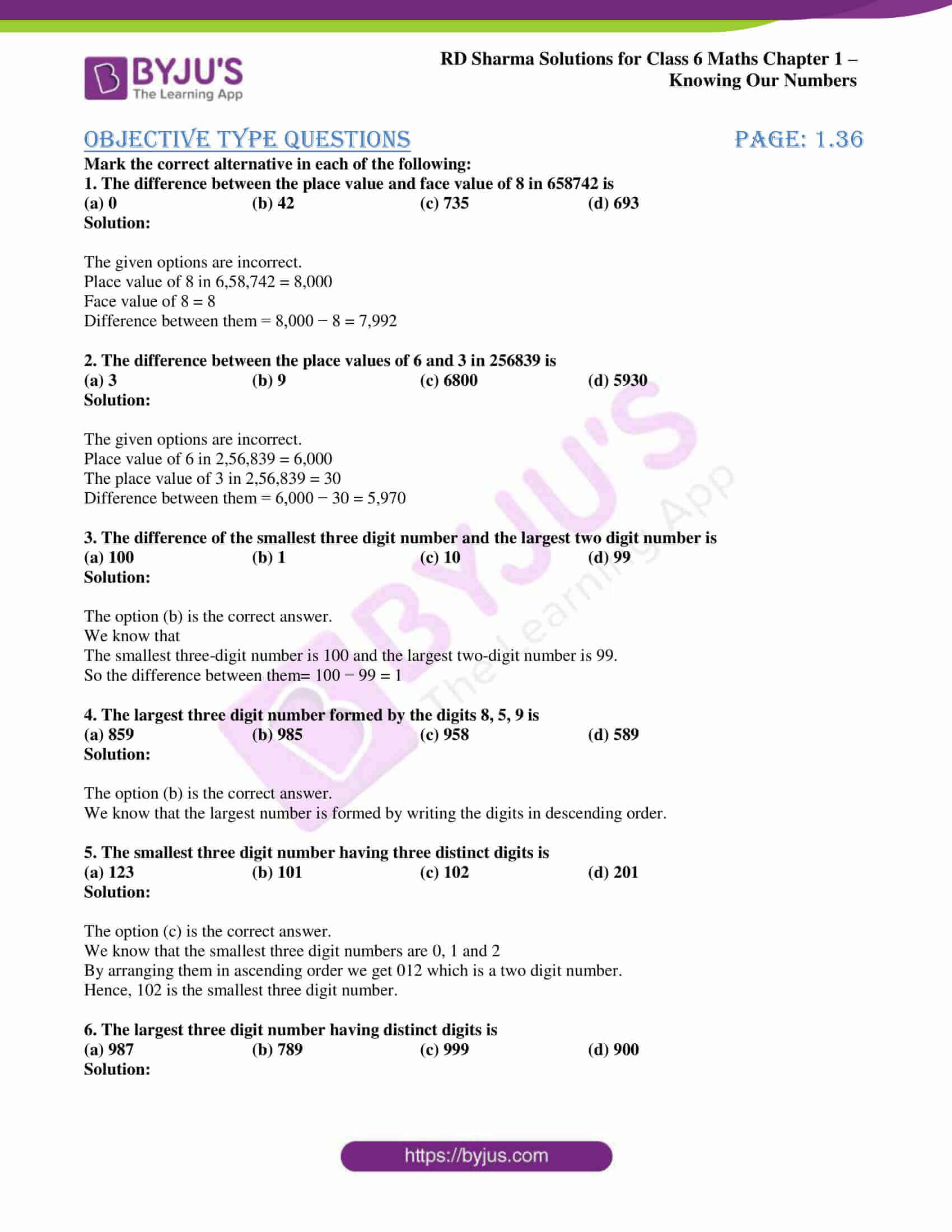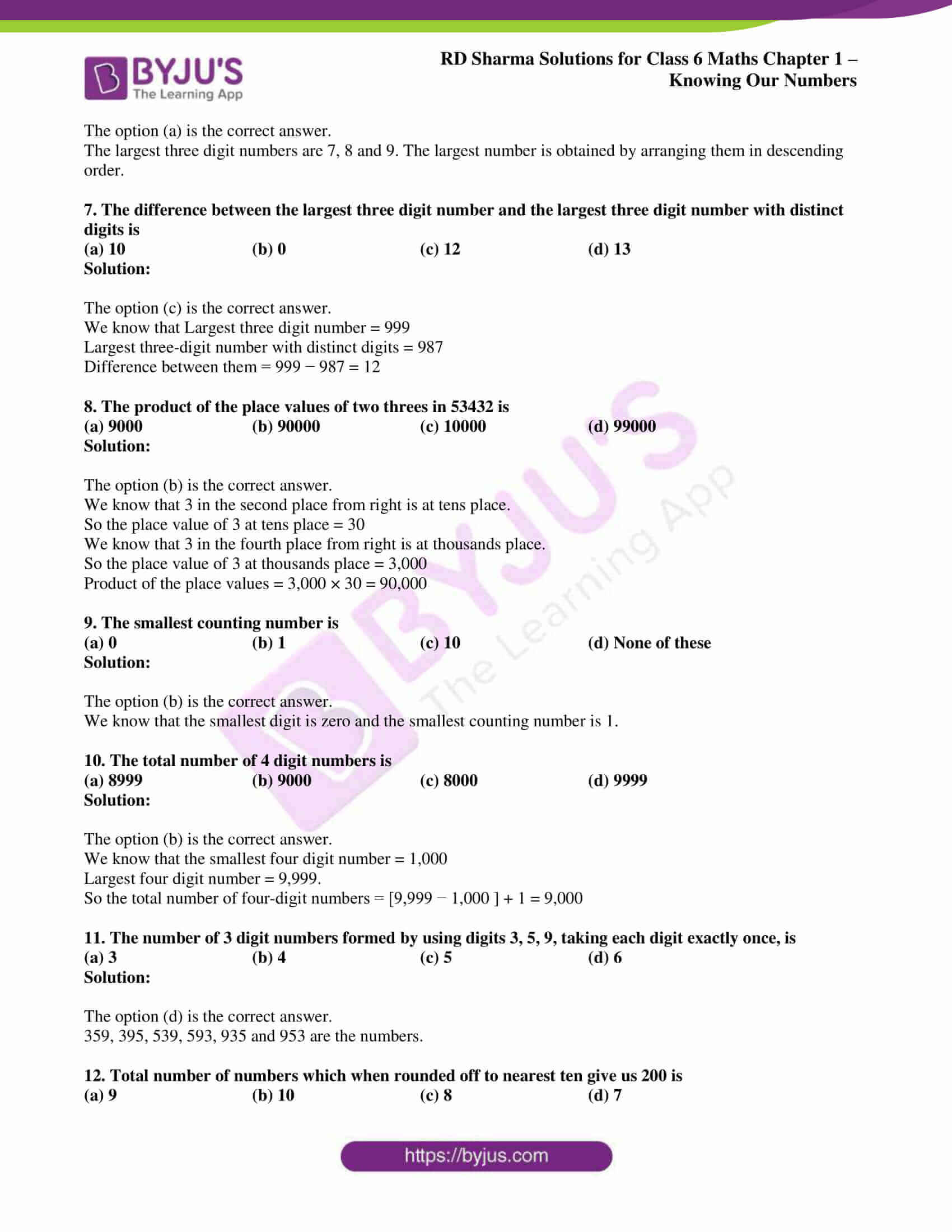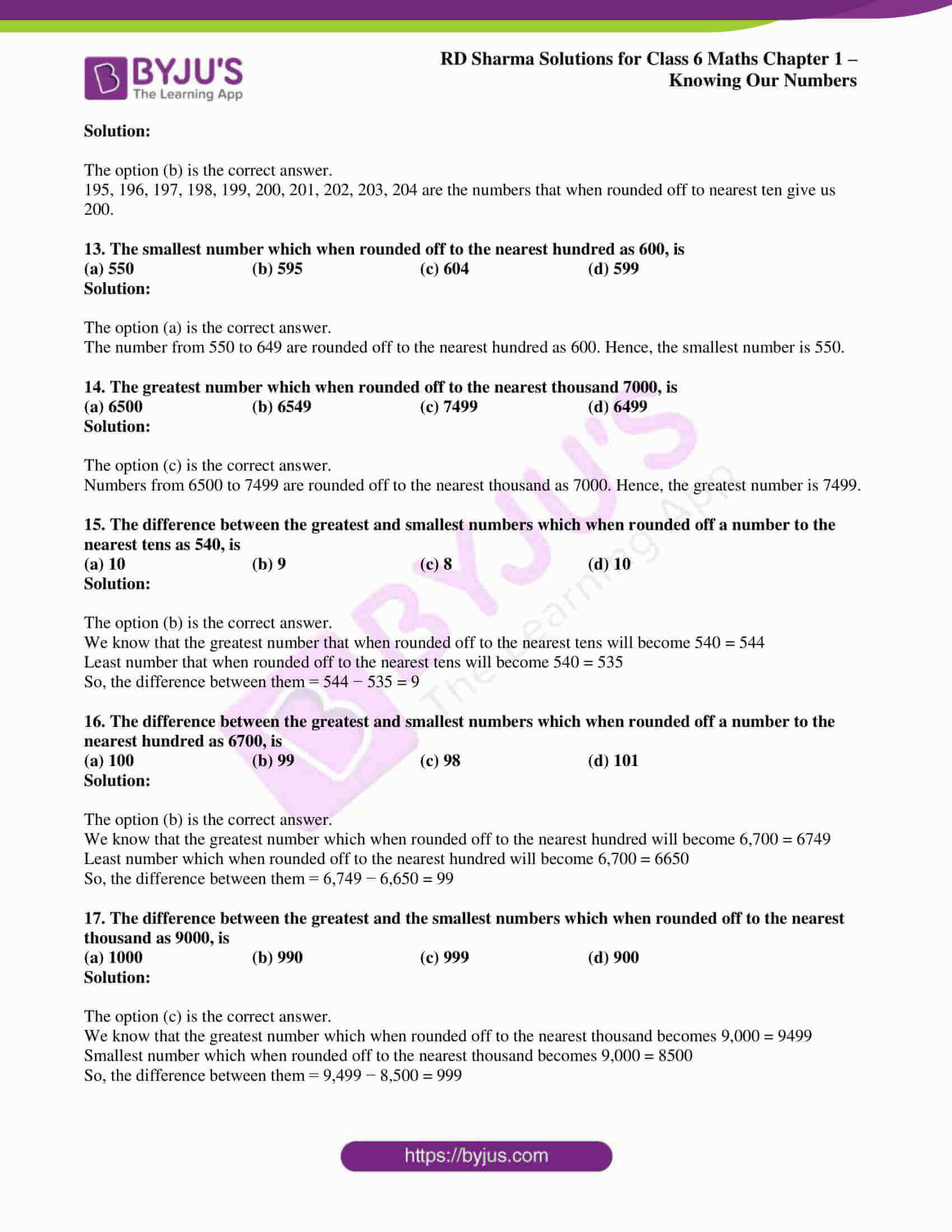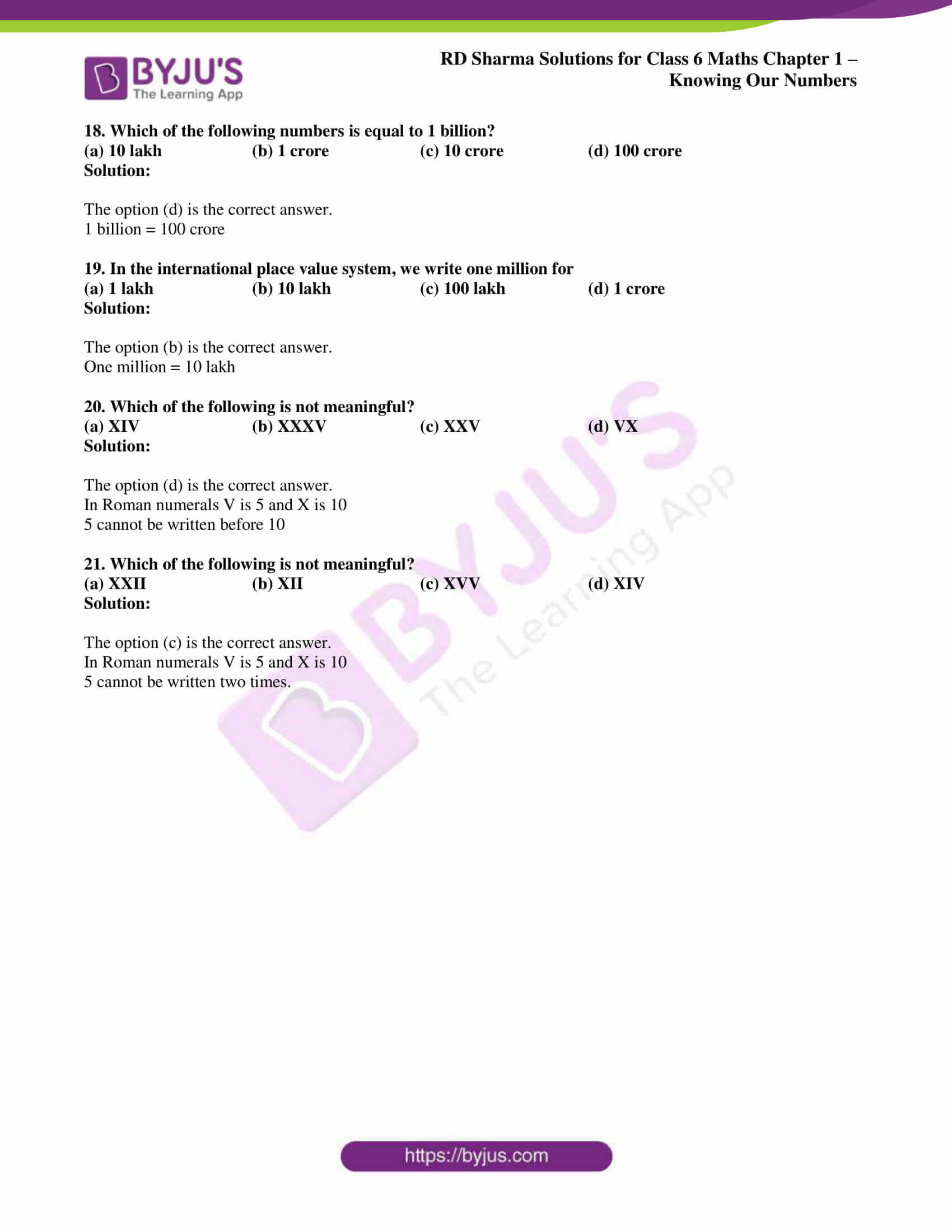# RD Sharma Solutions for Class 6 Maths Chapter 1: Knowing Our Numbers Objective Type Questions

A subject like Mathematics is a boon to the students to score well in the Class 6 exam. This Chapter mainly helps the students gain a better idea about numbers, which are important in our day to day lives. The students can make use of the RD Sharma solutions for Class 6 designed by subject matter experts to score well in the exam. The objective type questions available at the end of this Chapter cover major topics which are important from the exam point of view. RD Sharma Solutions for Class 6 Maths Chapter 1 Knowing Our Numbers Objective Type Questions PDF are provided here.

## RD Sharma Solutions for Class 6 Maths Chapter 1: Knowing Our Numbers Objective Type Questions Download PDF### Access answers to Maths RD Sharma Solutions for Class 6 Chapter 1: Knowing Our Numbers Objective Type Questions

Mark the correct alternative in each of the following:

1. The difference between the place value and face value of 8 in 658742 is

(a) 0 (b) 42 (c) 735 (d) 693

Solution:

The given options are incorrect.

Place value of 8 in 6,58,742 = 8,000

Face value of 8 = 8

Difference between them = 8,000 − 8 = 7,992

2. The difference between the place values of 6 and 3 in 256839 is

(a) 3 (b) 9 (c) 6800 (d) 5930

Solution:

The given options are incorrect.

Place value of 6 in 2,56,839 = 6,000

The place value of 3 in 2,56,839 = 30

Difference between them = 6,000 − 30 = 5,970

3. The difference of the smallest three digit number and the largest two digit number is

(a) 100 (b) 1 (c) 10 (d) 99

Solution:

The option (b) is the correct answer.

We know that

The smallest three-digit number is 100 and the largest two-digit number is 99.

So the difference between them= 100 − 99 = 1

4. The largest three digit number formed by the digits 8, 5, 9 is

(a) 859 (b) 985 (c) 958 (d) 589

Solution:

The option (b) is the correct answer.

We know that the largest number is formed by writing the digits in descending order.

5. The smallest three digit number having three distinct digits is

(a) 123 (b) 101 (c) 102 (d) 201

Solution:

The option (c) is the correct answer.

We know that the smallest three digit numbers are 0, 1 and 2

By arranging them in ascending order we get 012 which is a two digit number.

Hence, 102 is the smallest three digit number.

6. The largest three digit number having distinct digits is

(a) 987 (b) 789 (c) 999 (d) 900

Solution:

The option (a) is the correct answer.

The largest three digit numbers are 7, 8 and 9. The largest number is obtained by arranging them in descending order.

7. The difference between the largest three digit number and the largest three digit number with distinct digits is

(a) 10 (b) 0 (c) 12 (d) 13

Solution:

The option (c) is the correct answer.

We know that Largest three digit number = 999

Largest three-digit number with distinct digits = 987

Difference between them = 999 − 987 = 12

8. The product of the place values of two threes in 53432 is

(a) 9000 (b) 90000 (c) 10000 (d) 99000

Solution:

The option (b) is the correct answer.

We know that 3 in the second place from right is at tens place.

So the place value of 3 at tens place = 30

We know that 3 in the fourth place from right is at thousands place.

So the place value of 3 at thousands place = 3,000

Product of the place values = 3,000 × 30 = 90,000

9. The smallest counting number is

(a) 0 (b) 1 (c) 10 (d) None of these

Solution:

The option (b) is the correct answer.

We know that the smallest digit is zero and the smallest counting number is 1.

10. The total number of 4 digit numbers is

(a) 8999 (b) 9000 (c) 8000 (d) 9999

Solution:

The option (b) is the correct answer.

We know that the smallest four digit number = 1,000

Largest four digit number = 9,999.

So the total number of four-digit numbers = [9,999 − 1,000 ] + 1 = 9,000

11. The number of 3 digit numbers formed by using digits 3, 5, 9, taking each digit exactly once, is

(a) 3 (b) 4 (c) 5 (d) 6

Solution:

The option (d) is the correct answer.

359, 395, 539, 593, 935 and 953 are the numbers.

12. Total number of numbers which when rounded off to nearest ten give us 200 is

(a) 9 (b) 10 (c) 8 (d) 7

Solution:

The option (b) is the correct answer.

195, 196, 197, 198, 199, 200, 201, 202, 203, 204 are the numbers that when rounded off to nearest ten give us 200.

13. The smallest number which when rounded off to the nearest hundred as 600, is

(a) 550 (b) 595 (c) 604 (d) 599

Solution:

The option (a) is the correct answer.

The number from 550 to 649 are rounded off to the nearest hundred as 600. Hence, the smallest number is 550.

14. The greatest number which when rounded off to the nearest thousand 7000, is

(a) 6500 (b) 6549 (c) 7499 (d) 6499

Solution:

The option (c) is the correct answer.

Numbers from 6500 to 7499 are rounded off to the nearest thousand as 7000. Hence, the greatest number is 7499.

15. The difference between the greatest and smallest numbers which when rounded off a number to the nearest tens as 540, is

(a) 10 (b) 9 (c) 8 (d) 10

Solution:

The option (b) is the correct answer.

We know that the greatest number that when rounded off to the nearest tens will become 540 = 544

Least number that when rounded off to the nearest tens will become 540 = 535

So, the difference between them = 544 − 535 = 9

16. The difference between the greatest and smallest numbers which when rounded off a number to the nearest hundred as 6700, is

(a) 100 (b) 99 (c) 98 (d) 101

Solution:

The option (b) is the correct answer.

We know that the greatest number which when rounded off to the nearest hundred will become 6,700 = 6749

Least number which when rounded off to the nearest hundred will become 6,700 = 6650

So, the difference between them = 6,749 − 6,650 = 99

17. The difference between the greatest and the smallest numbers which when rounded off to the nearest thousand as 9000, is

(a) 1000 (b) 990 (c) 999 (d) 900

Solution:

The option (c) is the correct answer.

We know that the greatest number which when rounded off to the nearest thousand becomes 9,000 = 9499

Smallest number which when rounded off to the nearest thousand becomes 9,000 = 8500

So, the difference between them = 9,499 − 8,500 = 999

18. Which of the following numbers is equal to 1 billion?

(a) 10 lakh (b) 1 crore (c) 10 crore (d) 100 crore

Solution:

The option (d) is the correct answer.

1 billion = 100 crore

19. In the international place value system, we write one million for

(a) 1 lakh (b) 10 lakh (c) 100 lakh (d) 1 crore

Solution:

The option (b) is the correct answer.

One million = 10 lakh

20. Which of the following is not meaningful?

(a) XIV (b) XXXV (c) XXV (d) VX

Solution:

The option (d) is the correct answer.

In Roman numerals V is 5 and X is 10

5 cannot be written before 10

21. Which of the following is not meaningful?

(a) XXII (b) XII (c) XVV (d) XIV

Solution:

The option (c) is the correct answer.

In Roman numerals V is 5 and X is 10

5 cannot be written two times.